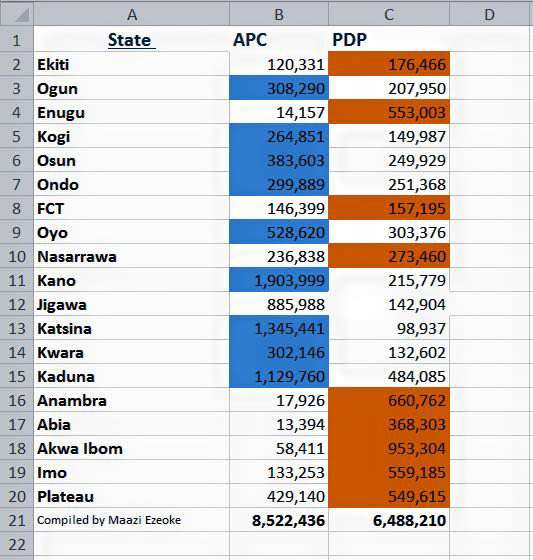0
917These are the results released so far by INEC in the 2015 presidential race in Nigeria. Figures here represent results so far for the two major parties- ruling Peoples’ Democratic Party (PDP) and All Progressives Congress.

Professor Attahiru Jega of INEC says the electoral body will resume announcement of results at 10 am tomorrow.

So far, Buhari leads in 10 states while Jonathan leads in 8, including Abuja, the capital of Nigeria.

Here are the raw scores:

1. Ekiti State
APC = 120,331
PDP = 176,466

2. Ogun State
APC = 308,290
PDP = 207,950

3. Enugu State
APC = 14,157
PDP = 553,003

4. Kogi State
APC = 264,851
PDP = 149,987

5. Osun State
APC = 383,603
PDP = 249,929

6. Ondo State
APC = 299,889
PDP = 251,368

7. Abuja
APC = 146,399
PDP = 157,195

8. Oyo State
APC = 528,620
PDP = 303,376

9. Nassarawa State
APC = 236,838
PDP = 273,460

10. Kano State
APC = 1,903,999
PDP = 215,779

11. Jigawa State
APC = 885,988
PDP = 142,904

12. Kastina State
APC = 1,345,441
PDP = 98,937

13.Kwara State
APC = 302,146
PDP = 132,602

APC = 1,127,760
PDP = 484,085

15. Anambra State
APC = 17,926
PDP = 660,762

16. Abia State
APC = 13,394
PDP = 368,303

17. Akwa-Ibom State
APC = 58,411
PDP = 953,304

18. Imo State
APC = 133,253
PDP = 559,185

19. Plateau State
APC = 429,140
PDP = 549,615